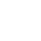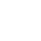# A brief look at infinity

What is infinity?

If the first thing that came to your mind was the amount of chocolate you'd like to eat or the number of weeks you'd like to have of summer vacation, then you are on the right track.

But if you thought of a very big number  a million, a trillion, a quadrillion  then we need to have a little talk.

Infinity is not the same thing as "bigness." It is above and beyond "big." A big number is no more infinite than a small number. Five million is no more infinite than five. Why? Because both are finite (FYE-nite). They are a certain amount, and no more.

As mathematicians define it, infinity is more like a summer vacation that never ends. In an infinite vacation, you would go to bed each night knowing that you'd have at least one more day off.

Infinity is a thing (let's avoid the word "number" for the moment) that is bigger than any number. It is bigger than 5, bigger than 500, bigger than 5 million. We represent that thing by the mathematical symbol infinity. (The man who came up with that symbol is described below.)

There is an infinity of counting numbers (the counting numbers are 1,2,3, and so on). If you try to reach the limit of the counting numbers, you can't. There's always one more, and one more after that. Even if you count up to one google (a google is the number 1 followed by 100 zeros), you will find that there is a number beyond it (a google and one) and a second one close on its heels (a google and two), and on and on.

We can use the infinity symbol in arithmetic, but the arithmetic of infinity is weird. For example:

infinity + 1 = infinity

Why is this? Because we know that infinity is bigger than any number. Adding 1 to infinity cannot change that property of infinity.

It is also true that:

infinity  1 = infinity

Again, when you take 1 away from infinity, the result is still larger than any limit. That's what infinity means: Something that's not finite, not limited. As applied to numbers, it means "very big" as well as "very small"  and everything else, too.

The truly weird part occurs when you subtract infinity from infinity. You can get any result you please! (Just the kind of question you'd like to have on math test, don't you think?) You can decide the correct answer because all answers are correct. You can make:

infinity  infinity = 0

You can also make it equal to 1 or 7 or 19.

Here's how: Let's return to the counting numbers (1,2,3, etc.). If you get rid of all of them, you will have nothing. Therefore:

infinity  infinity = 0

However, you might decide to subtract all the counting numbers starting with 8, leaving seven numbers (1, 2, 3, 4, 5, 6, and 7). You have removed an infinity of counting numbers, so you the result is:

infinity  infinity = 7

But the strangest result of all is:

infinity  infinity = infinity

How could that be? Again, start with the counting numbers. There are an infinite number of even numbers: 2, 4, 6, 8, and so on. (If you don't believe me, just try to think of an upper limit for the even numbers. You can't; there isn't one.) If you start with the counting numbers, which are infinite, and remove just the even numbers, which are also infinite, you are left with the odd numbers, which are  that's right  infinite. Therefore, you can correctly state that:

infinity  infinity = infinity

Think of it this way: Infinite does not mean "every number that exists." There are, in fact, an infinite number of infinite numbers. Infinity can be small, too. Start with the numeral 1, for example Divide it in half. Divide it in half again (1/4). And again (1/8). Keep going. What will you find? That there are an infinite number of fractions between 1 and 0.

For another strange example of "transfinite arithmetic" (that is, the arithmetic of infinity), read about the circle-and-line diagram on the next page.

The concept of infinity undergirds a lot of modern mathematics, even practical mathematics. For example: Suppose you are stretching a clothesline across your backyard. You want to know how tightly you need to stretch it to prevent it from sagging under the weight of wet laundry. The easiest way to analyze this problem mathematically is to pretend that the clothesline is made up of an infinite number of pieces, each of them infinitesimal (that is, infinitely short) in length. This analysis produces a very simple (well, for a mathematician) equation to describe the curve of the line.

We should end our discussion of infinity with English poet William Blake. Blake has perhaps the most famous statement about infinity in his poem "Auguries of Innocence," written about 1801:

To see a world in a grain of sand

And a heaven in a wild flower,

Hold infinity in the palm of your hand

And eternity in an hour.

Our hands are relatively small things. They can hold only a few pieces of chocolate or grasp a small number of coins. But they can mark today as vacation. And tomorrow. And the day after that. And so we can continue reaching toward infinity.

Which is bigger?

Look at the two geometric figures pictured above, a circle and an infinite line. Which one is bigger?

Most people would say that the infinite line is bigger. It's longer than the circle. But mathematicians see it another way. They can prove that, for every point on the infinite line, there is a corresponding point on the circle. And for every point on the circle, there is a corresponding point on the line. In mathematical terms, then, a circle is just an infinite line that's been scrunched up into a ball.

Remember, we're talking about an ideal circle and line  figures not drawn with pencil, ruler, and compass. The points on these figures are as small as they need to be. Infinitely small, in fact.

Look at the diagram. The circle touches the line at only one point, as if it were the sun sitting on the horizon at sunset. Point A is directly opposite the point where the circle touches the line. From any point you choose on the line (B, C, D, E), draw a line through point A.

Any line you draw crosses the circle at exactly one point before it hits Point A. If you're a mathematician, you're well on your way to proving that for each point on the infinite line there is a corresponding point on the circle, and vice versa. (We can't explain the proof here  it has to do with the unique angles described by the lines drawn through Point A.) But that fact is enough to prove that the circle is as infinite as the line.

A sideways symbol

The concept of infinity was known to the ancient Greeks, though Aristotle found the idea somewhat suspect. He called it "imperfect, unfinished, unlimited, and therefore unthinkable."

The symbol for infinity, an elongated figure 8 lying on its side (infinity), was invented by English mathematician John Wallis (1616-1703), shown here. Wallis first used the symbol in a book titled "Arithmetica Infinitorum" ("The Arithmetic of Infinitesimals") in 1655. He was probably the best-known English mathematician before Sir Isaac Newton (1642-1727) of falling-apple fame.

Wallis was also a cryptographer, a maker and breaker of codes. He decoded messages for pro-Parliamentarian rebels during the English Civil Wars (1642-51).

You've read  of  free articles. Subscribe to continue.https://www.csmonitor.com/2002/0924/p18s03-hfks.htmlhttps://www.csmonitor.com/subscribe

## Subscription expired

Your subscription to The Christian Science Monitor has expired. You can renew your subscription or continue to use the site without a subscription.

This message will appear once per week unless you renew or log out.

## Session expired

Your session to The Christian Science Monitor has expired. We logged you out.